# Test: Electrical & Electronic Measurements- 7

## 10 Questions MCQ Test RRB JE for Electrical Engineering | Test: Electrical & Electronic Measurements- 7

Description
Attempt Test: Electrical & Electronic Measurements- 7 | 10 questions in 30 minutes | Mock test for Electrical Engineering (EE) preparation | Free important questions MCQ to study RRB JE for Electrical Engineering for Electrical Engineering (EE) Exam | Download free PDF with solutions
QUESTION: 1

### A voltage signal V(t) = A + B sin ωt is fed to an oscilloscope with the coupling mode set to DC, other settings being appropriate. The displayed wave can be expressed as

Solution:

When a voltage signal is fed to an oscilloscope with DC coupling entire waveform will be displayed including the DC component.

QUESTION: 2

### Calculate the ratio of vertical to horizontal frequencies for an oscilloscope which display the following Lissajous figure.Solution:

Ratio of the two frequencies is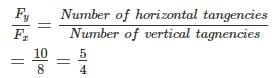*Answer can only contain numeric values
QUESTION: 3

### A sinusoidal waveform, when observed on an oscilloscope, has a peak to peak amplitude of 4 cm. If the vertical sensitivity setting is 7.5 V/cm, the rms value of the voltage is__ (in V)

Solution:

Vertical voltage sensitivity = 7.5 V/cm
Peak to peak voltage = 7.5 × 4 = 30 V
Maximum voltage = 15 V
Rms value of the voltage = 15/√2 = 10.6 V

QUESTION: 4

A single channel analog oscilloscope is operated in X-Y mode. An ellipse rotating in anticlockwise direction and having major axis in 2nd and 4thquadrants displayed on the screen. The peak Y deflection is at 5 divisions and Y intercept is at 3 divisions. The phase difference between both the input signals is ______

Solution: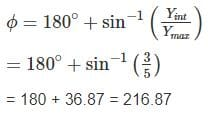QUESTION: 5

An electrostatically deflected CRT has plane parallel deflecting plates which are 2.5 cm long and 0.5 cm apart. The distance of the screen form the center of the plates is 20 cm. The accelerating voltage is 2500 V. The deflecting voltage required to get the corresponding deflection of 4 cm on the screen and the velocity of the electron beam entering the field respectively are.

Solution:

Given that y = 4 cm
D = 2.5 cm
L = 20 cm
d = 0.5 cm
Va = 2500 V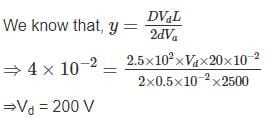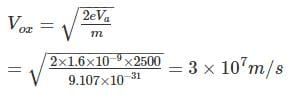QUESTION: 6

In an analog single channel cathode ray oscilloscope (CRO), the x and y sensitivities are set as 1ms/div. and 1V/div. respectively. The y-inputs is connected to a voltage signal 4 cos (200 πt – 45°) V. The trigger source is internal, level chosen is zero and the slope is positive. The display seen on the CRO screen is

Solution:

Input = 4 cos (200 πt – 45°)
Peak voltage = 4 V
Y sensitivity = 1V/div
No of divisions on y – axis = 4/1 = 4
ω = 200π
2π f = 200π
⇒ T = 1/100 = 10 ms
X sensitivity = 1ms/div
No. of x – divisions = 10/1 = 10 for one full cycle
The trigger is set to zero
Hence waveform starts from zero.

QUESTION: 7

The input impedance of a CRO is equivalent to a 1 MΩ resistance in parallel with a 45 pF capacitance. It is used with a compensated 10 to 1 attenuation probe. The effective input capacitance at the probe tip is

Solution: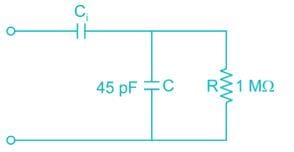Given that 10 to 1 attenuation probe.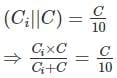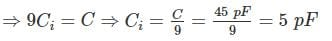Effective input capacitance = (45 || 5)
= 4.5 pF

*Answer can only contain numeric values
QUESTION: 8

A Lissajous pattern on the oscilloscope is stationary having 8 vertical maximum values and 6 horizontal maximum values. The frequency of vertical input of the frequency of horizontal input if the frequency of horizontal input is 1800 kHz is – (in kHz)

Solution:

Given that,
Horizontal frequency (fH) = 1800 kHz
Number of vertical tangencies (Nv) = 8
Number of horizontal tangencies (NH) = 6
We know that,
fHNH = fvNv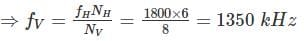*Answer can only contain numeric values
QUESTION: 9

The below given ellipse is displayed on the screen when a CRO is operated in x-y display mode. The phase difference between both input signals is – (in degree)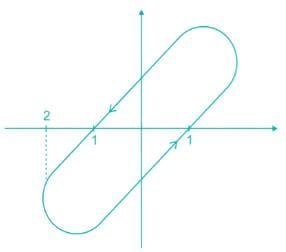Solution: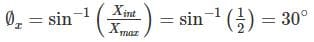f = 360°  - fx = 330°
[∵ since rotation is anticlockwise]

*Answer can only contain numeric values
QUESTION: 10

A CRO has an anode voltage 3000 V and parallel deflection plate 3 cm long and 7 mm apart. The screen is 35 cm from the centre of the plates. Find the input voltage required to deflect the beam through 4 cm. The input voltage is applied to the deflecting plates through amplifiers having an overall gain of 100.

Solution: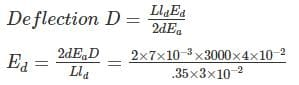= 160 V
∴ Input voltage required = 160/100 = 1.6 VUse Code STAYHOME200 and get INR 200 additional OFF Use Coupon Code